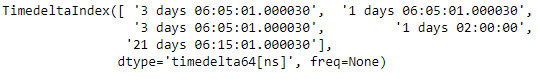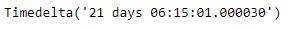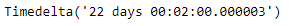Related Articles
Python | Pandas TimedeltaIndex.min
• Last Updated : 28 Dec, 2018

Python is a great language for doing data analysis, primarily because of the fantastic ecosystem of data-centric python packages. Pandas is one of those packages and makes importing and analyzing data much easier.

Pandas` TimedeltaIndex.min()` function return the minimum value of the TimedeltaIndex object or minimum along an axis.

Syntax : TimedeltaIndex.min(axis=None, *args, **kwargs)

Parameters : None

Return : Timedelta object

Example #1: Use `TimedeltaIndex.min()` function to find the minimum value in the given TimedeltaIndex object.

 `# importing pandas as pd``import` `pandas as pd`` ` `# Create the TimedeltaIndex object``tidx ``=` `pd.TimedeltaIndex(data ``=``[``'3 days 06:05:01.000030'``, ``'1 days 06:05:01.000030'``,``                                ``'3 days 06:05:01.000030'``, ``'1 days 02:00:00'``, ``                                                 ``'21 days 06:15:01.000030'``])`` ` `# Print the TimedeltaIndex object``print``(tidx)`

Output :Now we will use the `TimedeltaIndex.min()` function to find the minimum value in tidx object.

 `# find minimum value``tidx.``min``()`

Output :As we can see in the output, the `TimedeltaIndex.min()` function has returned the minimum value in the given TimedeltaIndex object.

Example #2: Use `TimedeltaIndex.min()` function to find the minimum value in the given TimedeltaIndex object.

 `# importing pandas as pd``import` `pandas as pd`` ` `# Create the TimedeltaIndex object``tidx ``=` `pd.TimedeltaIndex(data ``=``[``'06:05:01.000030'``, ``'3 days 06:05:01.000030'``,``                                ``'22 day 2 min 3us 10ns'``, ``'+23:59:59.999999'``,``                             ``'13 days 06:05:01.000030'``, ``'+12:19:59.999999'``])`` ` `# Print the TimedeltaIndex object``print``(tidx)`

Output :Now we will use the `TimedeltaIndex.min()` function to find the minimum value in tidx object.

 `# find minimum value``tidx.``min``()`

Output :As we can see in the output, the `TimedeltaIndex.min()` function has returned the minimum value in the given TimedeltaIndex object.

Attention geek! Strengthen your foundations with the Python Programming Foundation Course and learn the basics.

To begin with, your interview preparations Enhance your Data Structures concepts with the Python DS Course.

My Personal Notes arrow_drop_up